How Cheenta works to ensure student success?
Explore the Back-Story
Get inspired by the success stories of our students in IIT JAM MS, ISI  MStat, CMI MSc DS.  Learn More

# How to Measure the Length of your Earphone from a Pic?| Cheenta Probability SeriesThis is our 5th post in the Cheenta Probability Series. This article teaches how to mathematically find the length of an earphone wire by its picture.

Let's explore some truths.

• A Line is made up of Points.
• A Curve is made up of Lines.
• Earphones are as Messy.

This article is all about connecting these three truths to a topic in Probability Theory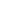Geometry, which is not so famous among peers, yet its' usefulness has led one to make an app in Google Play Store, which has only one download (by me). The sarcasm is towards the people, who didn't download. (obviously).

Today is the day for Cauchy Crofton's Formula, which can measure the length of any curve using random straight lines by counting points. It is all encoded in the formula.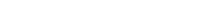This is the begining of a topic called Integral Geometry.

This formula intuitively speaks the truth

The Length of a Curve is the Expected Number of Times a "Random" Line Intersects it.

## Describing a Line (like seriously?).

I mean a "Random" Line.

Thus the Space of all Lines in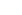can be represented in "kinda" polar coordinates S = {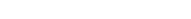} where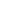are as indicated.

Now, we are now uniformly selecting this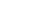over S. This is how we select a "Random" Line.

## Understanding Crofton's Gibberish

If C is the curve, whose length we are interested to measure. Then,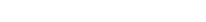.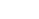= The Length of the curve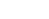= The Number of times "Random Line" corresponding tointersects C.

Crofton meant the following on a pretty, differentiable, and cute (regular) curve C that: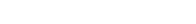## The First Truth

Here, we will find the length of a Line using "Random Lines". We will see, how counting points on the line, can help us find the length of a line.

Here, we choose C = Line.

A Line intersects another Line exactly once.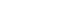. Hence,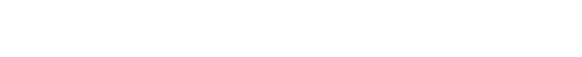## The Second Truth

Approximate Curves by Lines.

Refer to Approximation of Curves by Line Segments by Henry Stone.

## Just Limit!

Now, take apply the result on these lines. By linearity of expectation and the uniform convergence of the Lines to the Curve, we get the same for the curve.

Suppose that the curve c is parameterized on the interval [a,b]. Define a partition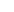to be a collection of points that subdivides the interval, say {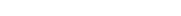}.

Define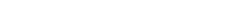Then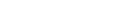Now, each of the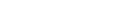are approximated by the Crofton's Formula. Now, taking the limit gives ust eh required result. QED.

## Measuring the Length of an Earphone

Create a Mesh of Lines (Coordinate System) - A Fixed Sample representing the Population of the Random Lines. Then count the number of intersections using those mesh of lines only and add it up.

The mathematics of the above argument is as follows.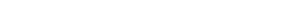An example is show below. This is done in the app named Cauchy-Crofton App, which unfortunately is not working now and I have reported.

## Future Articles and Work

There are lots of corollaries coming up like Isoperimetric Inequality, Barbier's Result, etc. I will discuss these soon. Till then,

Stay Tuned!

Stay Blessed!

## Subscribe to Cheenta at Youtube

This is our 5th post in the Cheenta Probability Series. This article teaches how to mathematically find the length of an earphone wire by its picture.

Let's explore some truths.

• A Line is made up of Points.
• A Curve is made up of Lines.
• Earphones are as Messy.

This article is all about connecting these three truths to a topic in Probability TheoryGeometry, which is not so famous among peers, yet its' usefulness has led one to make an app in Google Play Store, which has only one download (by me). The sarcasm is towards the people, who didn't download. (obviously).

Today is the day for Cauchy Crofton's Formula, which can measure the length of any curve using random straight lines by counting points. It is all encoded in the formula.This is the begining of a topic called Integral Geometry.

This formula intuitively speaks the truth

The Length of a Curve is the Expected Number of Times a "Random" Line Intersects it.

## Describing a Line (like seriously?).

I mean a "Random" Line.

Thus the Space of all Lines incan be represented in "kinda" polar coordinates S = {} whereare as indicated.

Now, we are now uniformly selecting thisover S. This is how we select a "Random" Line.

## Understanding Crofton's Gibberish

If C is the curve, whose length we are interested to measure. Then,.= The Length of the curve= The Number of times "Random Line" corresponding tointersects C.

Crofton meant the following on a pretty, differentiable, and cute (regular) curve C that:## The First Truth

Here, we will find the length of a Line using "Random Lines". We will see, how counting points on the line, can help us find the length of a line.

Here, we choose C = Line.

A Line intersects another Line exactly once.. Hence,## The Second Truth

Approximate Curves by Lines.

Refer to Approximation of Curves by Line Segments by Henry Stone.

## Just Limit!

Now, take apply the result on these lines. By linearity of expectation and the uniform convergence of the Lines to the Curve, we get the same for the curve.

Suppose that the curve c is parameterized on the interval [a,b]. Define a partitionto be a collection of points that subdivides the interval, say {}.

DefineThenNow, each of theare approximated by the Crofton's Formula. Now, taking the limit gives ust eh required result. QED.

## Measuring the Length of an Earphone

Create a Mesh of Lines (Coordinate System) - A Fixed Sample representing the Population of the Random Lines. Then count the number of intersections using those mesh of lines only and add it up.

The mathematics of the above argument is as follows.An example is show below. This is done in the app named Cauchy-Crofton App, which unfortunately is not working now and I have reported.

## Future Articles and Work

There are lots of corollaries coming up like Isoperimetric Inequality, Barbier's Result, etc. I will discuss these soon. Till then,

Stay Tuned!

Stay Blessed!

## Subscribe to Cheenta at Youtube

This site uses Akismet to reduce spam. Learn how your comment data is processed.

### Knowledge Partner